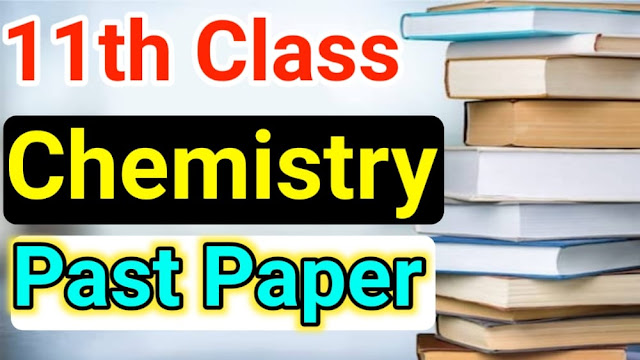# Chemistry Past Paper 2021 1st year Lahore board

## Chemistry Past Paper 2021 1st year Lahore board

Dear students here you will see Chemistry Past Paper 2021 1st year Lahore board. You can read complete and also learn from online our site. We are always here to help 1st-year students and all schools and colleges and universities students. Please read this Chemistry Past Paper 1st year Lahore board of 2021. If you like these past papers then you can share them on social media like WhatsApp. Twitter, Facebook and pinterst. Student if you want to find any other books solutions then please tell us which books solutions you need because our aim is to provide correct and accurate solutions to all students so that students could easily understand education in our country.Chemistry Past Paper 2021 1st year Lahore board - 11th Class

### We Also Recommended

1st-year Chemistry Most Important Short Questions For 2022

Lahore board past paper

past paper 2021 Lahore board

chemistry Lahore board paper

### 1st Year Chemistry Past Paper MCQs 2021

Which of the following is a pseudo solid?

Glass

The number of bonds in nitrogen molecules is

Two @ and one Pi Bond

The temperature of the natural plasma is about:

20000C

18 g glucose is dissolved in 90 g of water, the relative

lowering of vapor pressure is:

1/51

1-calorie is equivalent to:

4.184j

Nickel has a number of Isotopes:

5

PH of human blood is:

7.35

Bond angles @ = Gama = 90 degrees: B not equal and axes a b and c not equal for crystal system:

Monoclinic

1 gram formula of NaCl is equal to :

58.5g

Solvent extraction is an equilibrium process and it is controlled by:

Distribution Law

the de-Broglie equation is represented as:

lamda = h/mv

The stronger the oxidizing agent, the greater is the:

Reduction Potential

The veolicty of photon is:

Independent of waveolwength

Pressure remaining constant, at which temperature the

the volume of the gas will become twice what it is 0 C:

546 K

These above-solved MCQs are Lahore board 2021 past paper MCQs we hope these MCQs would be helpful for your in-class tests or final exams please read complete and try to learn all these MCQs.  Please don't skip this post now will start short questions of chemistry Past Paper 1st year Lahore board of 2021 - 11th Class.

### Short Q.1 of 11th Chemistry

• How is the Law of conservation of mass obeyed during stoichiometric calculation?

• Why do N2  and CO have the same number of electrons, protons, and neutrons? Justify

• Define mole. Calculate the gram atoms(moles) in 0.1g of sodium?

• Draw a beautiful diagram of the sublimation process?

• Write down the uses of the chromatography?

• What is the physical meaning of R?

• Prove boyl law in the light of K.M.T?

• What are the two characteristics of plasma?

• Write down the quantitative statement of Charles Law?

• Define heat of solution?

• Who will you justify that the lowering of vapor pressure is colligative property?

• Differentiate between ideal and non-ideal solutions?

### Short Q.2 of 1st year Chemistry

• Ethyl alcohol is soluble in water why?
• Explain H bonding in deoxyribonucleic acid [DNA]
• What do you know about anisotropy explain with example?
• What is entropy give n example?
• Write two nuclear reactions for the production of gamma radiations and  B-particles?
• Define defect of rutherford atomic Model?
• Define Heisenberg's uncertainty principle and write its mathematical equation?
• Write names of different quantum numbers?
• Write Henderson's equation for acidic and basic buffer.
• Why do we need a buffer solution?
• Explain specific rate constants briefly?
• What is the zero-order reaction? Give one example?

### Short Q.3 of 11th Class Chemistry

•  Define bond order and give one example?
• Draw diagram for the formation of bonding and antibonding molecular orbitals for H2 molecule
• Define sigma bond and pi bond
•   What is entropy give n example?
• Define atomic orbital hybridization
•  Explain specific rate constants briefly?
• What is the first law of thermodynamics give its mathematical equation?
• Define enthalpy of combustion ∆HAC+
• How anodized aluminum is prepared in an electrolytic cell?
• Define defect of rutherford atomic Model?
• Draw a diagram of standard hydrogen electrode(SHE)
• Define electrochemical series?

### Long Question of Chemistry Past Paper

• NH3 gas can be produced by heating together NH4CL and Ca(OH)2, if a mixture containing 100g of each solid is heated then calculate the number of grams of NH3 produced  2NH4CL+Ca(OH) Arrow Right Side  CaCL2 +2NH3 + 2H2O (Water)
• Explain isomorphism with examples?
• Give the postulates of kinetic Energy molecular theory?
• Derive an expression to determine the radius of orbits using the Bohr model?
• What is sp^2 hybridization, how ist explain the structure of ethene What is the Hess law? Explain by giving two examples?
• Calculate the pH of a buffer solution in which 0.11 molar CH3COONa and 0.09 molar acetic said solutions are present, Ka for the CH3COOH is 1.85 multiply 10^5.
• Define the half-life period. How the order of reaction can be determined by knowing the half-life of the reaction?
• What are the colligative properties of solutions? Explain the elevation of boiling points.
• Describe the construction and working of standard hydrogen electrodes.

1st-year Chemistry Most Important Short Questions For 2022

Lahore board past paper

past paper Lahore board

chemistry Lahore board paper

11th chemistry past paper Lahore board

Lahore board chemistry paper

11th chemistry past paper bise Lahore board

11th class chemistry past paper Lahore board